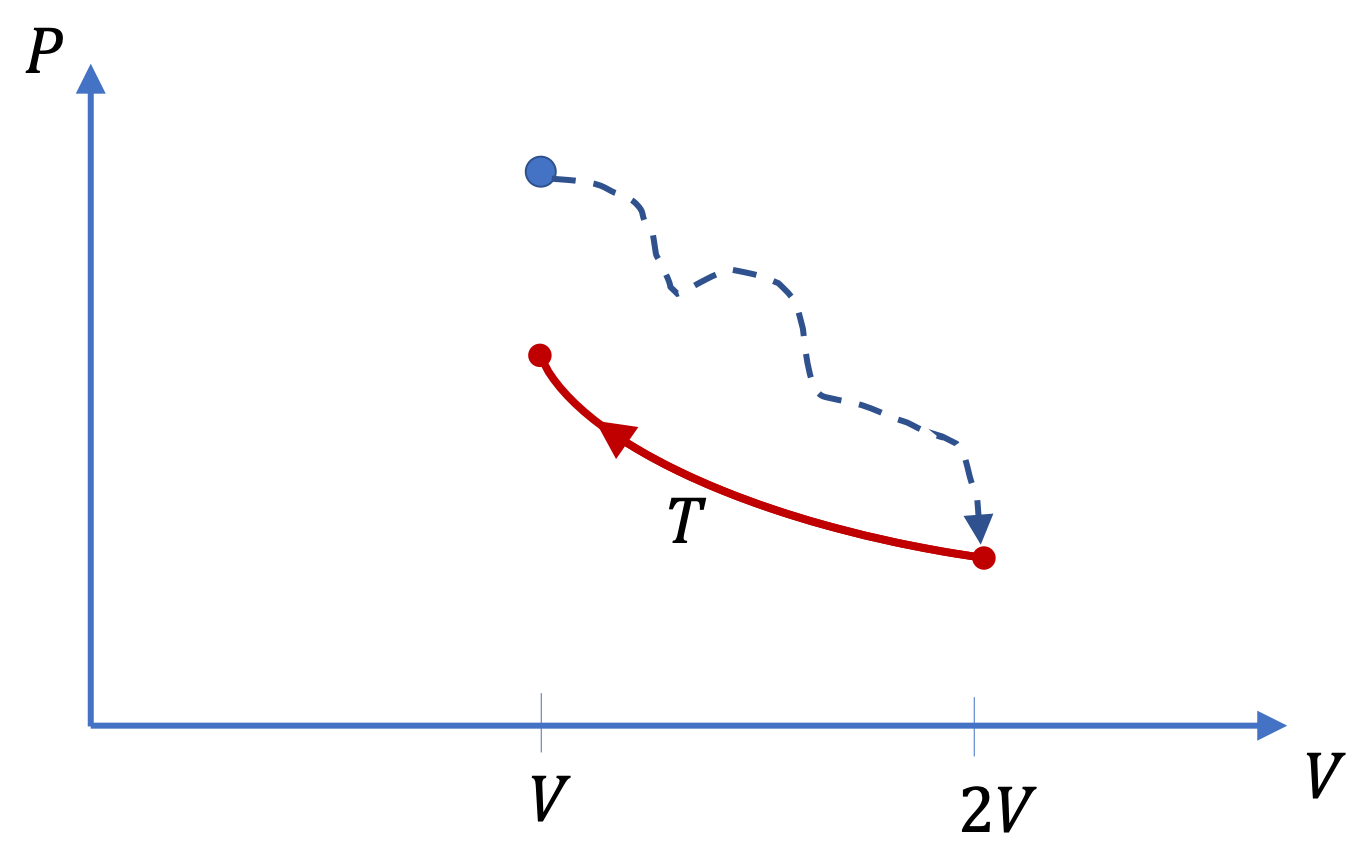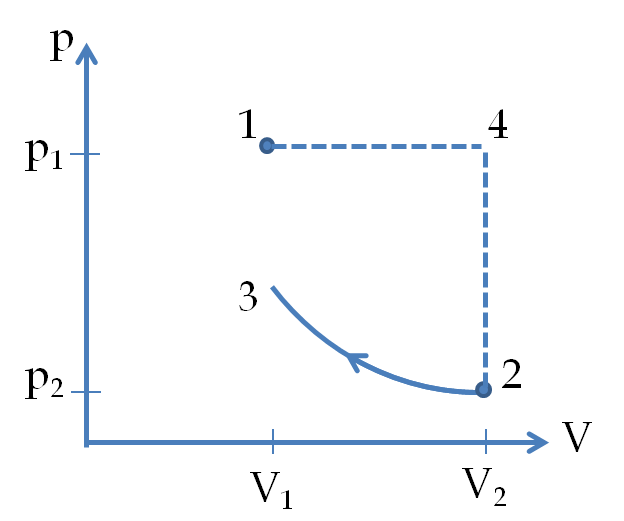## Section26.4Entropy Change in Irreversible Processes

The definition of entropy gives us only an inequality for irreversible processes. For an irreversible process that transforms a system from state A to state B, we have the following inequality.

\begin{equation} S(B) - S(A) \gt \left( \sum \dfrac{\Delta Q}{T} \right)_{\text{irr}}.\label{eq-entropy-inequality-in-irreversible-process}\tag{26.4.1} \end{equation}

The Calculus folks should read the sum as integral. Here the right side may not be calculable since we may not know $\Delta Q$ and/or $T$ during the process since the process is an irreversible process.

Even though Eq. (26.4.1) does not help us compute entropy, if we know the state A and B, then we can imagine a reversible process that will transform the system from state A to state B. We can use this imagined reversible process to find the entropy change.

\begin{equation} S(B) - S(A)=\left( \sum \frac{\Delta Q}{T} \right)_{\text{imagined rev process}}.\tag{26.4.2} \end{equation}

Since entropy is a state function, the difference of entropy between states A and B does not depend on how the change was made - whether the change was made by a reversible process or by an irreversible process. It should be the same for any process between A and B. Therefore, if the actual process performed between states A and B is an irreversible process, then, to find the entropy change, we can invent some convenient reversible process that starts with state A and ends at state B. The entropy change over the imagined reversible process will equal the entropy change over the irreversible process in question. I will now present two examples to make this point clearer.

A glass beaker of mass $600\text{g}$ contains $800\text{ g}$ of water at $20^{\circ}\text{C}\text{.}$ The beaker is put in a microwave and heated. At the end the temperature of water and beaker becomes $80^{\circ}\text{C}\text{.}$ What is the change in entropy of the beaker and water? Use specific heat $c = 0.2\text{ cal/g.K}$ for glass and $c = 1.0\text{ cal/g.K}$ for water.

Hint

Think of an easy-to-calculate reversible process between the initial and final states.

$171\text{ cal/K}$

Solution

We note that water is not heated slowly enough to be quasi-static and reversible. Therefore we have an irreversible process between two states: beaker+water at $20^{\circ}\text{C}$ (state A) and same at $80^{\circ}\text{C}$ (state B). We do not have any formula for directly evaluating entropy for this process.

We need to imagine a reversible process or processes that will cause state A to change to state B. One way this can be done is as follows. We can imagine an infinite number of heat baths of different temperatures between $20^{\circ}\text{C}$ and $80^{\circ}\text{C}\text{.}$ The system at temperature $T$ is then dipped into a bath at temperature $T+dT$ so that the temperature of the system rises by an infinitesimally amount quasi-statically and reversibly. The change of entropy in one such infinitesimal reversible process is

\begin{equation*} dS = \left(m c_p \frac{dT}{T} \right)_{beaker} + \left(m c_p \frac{dT}{T} \right)_{water} \end{equation*}

Integrating from $T=T_i$ to $T=T_f$ we find the following.

\begin{equation*} \Delta S = \left(m c_p \ln\frac{T_f}{T_i} \right)_\text{beaker} + \left(m c_p \ln\frac{T_f}{T_i} \right)_\text{water}. \end{equation*}

Now, putting in the values $T_i=20+273 = 293\text{K}$ and $T_f = 80+273=353\text{K}\text{,}$ along with other numerical values, we find the following.

\begin{equation*} \Delta S = 171\text{ cal/K}. \end{equation*}

Two moles of a monatomic ideal gas at $-50^{\circ}\text{C}$ is in one chamber of a two chamber insulated container where the chambers are equal in volume and are connected by a stopcock. The other chamber is evacuated to a perfect vacuum. When the stopcock is opened the gas rushes into the second chamber without any effort. Since gas does not move any piston or wall no work is done in the process. Find the entropy change.

Hint

$\Delta S \ne 0\text{.}$ Must imagine a reversible process that transforms the state. Think of an isothermal process.

$11.5\text{ J/K} \text{.}$

Solution

Even though no heat enters or exits the system, the entropy change is not necessarily zero. We cannot use $dQ/T$ of an irreversible process to find the entropy change. We have to imagine a reversible process between the initial and final state to carry out the calculation of entropy change.

Let us make sure we are clear about the initial and final states, A and B. Since no work is done and no heat is exchanged, the internal energy of the gas does not change. As internal energy of an ideal gas is only a function of temperature, this means that the temperature of the final state is same as that of the initial state.

\begin{align*} \amp \text{State } A:\ \ T=223\text{K}, \text{ volume } V, \text{ pressure } p_A.\\ \amp \text{State } B:\ \ T=223\text{K}, \text{ volume } 2V, \text{ pressure } p_B. \end{align*}

By using the ideal gas law, we can show that $p_B = p_A/2\text{.}$ Now we can imagine some convenient reversible processes for calculation of entropy. Since temperature of state B is same as that of state A, we can imagine an isothermal reversible process.

Imagined reversible process: Isothermal process between A and B. As the system is an ideal gas, the internal energy is only a function of temperature, which makes $dU = 0$ on this process. Therefore, from the first law of thermodynamics we get

\begin{equation*} dQ = pdV = \dfrac{nRT}{V}\, dV \end{equation*}

Hence the change in entropy is

\begin{equation*} \Delta S = nR\int_{V_A}^{V_B}\frac{dV}{V} = nR\ln\frac{V_B}{V_A} = 2\ mol\times \frac{8.31\ J}{mol. K}\ln(2) = 11.5\text{ J/K}. \end{equation*}

This says that even when $(\Delta Q)_\text{irr} = 0\text{,}$ $\Delta S\ne 0\text{.}$

Three moles of the Nitrogen gas at 400 K in a container is suddenly expanded to twice its volume. The process is so rapid that no heat is exchanged with the environment but the temperature of the final state upon reaching equilibrium is found to be 300 K. The gas is then compressed isothermally at 300 K back to the initial volume.Find the total change in entropy of the gas.

Data: Treat the Nitrogen gas as a diatomic ideal gas with the molar specific heat $C_V = \frac{5}{2} R\text{.}$

Hint

Replace the irreversible sudden expansion process by a constant pressure and a constant volume process.

$-17.9\ \text{J/K}\text{.}$

Solution

We redraw the processes in the following diagrma, where the origial irreversible process is now shown as 1-2, which will be replaced by a reversible process 1-4-2 for entropy calculations. For simplicity, I chosethe process 1-4-2 since it consists of constant pressure and constant volume steps for which entropy changes are easily computed.\begin{equation*} \Delta S_{12} = \Delta S_{14} + \Delta S_{42}. \end{equation*}

Since 1-4 process involved changing volume the heat will have both the change in the internal energy and the work.

\begin{align*} \Delta S_{14} \amp = \int \frac{dQ}{T} = \int \frac{dU}{T} + \int \frac{pdV}{T}\\ \amp = \int \frac{nC_V dT}{T} + \int \frac{n RdV}{V} = \int \frac{nC_V dV}{V} + \int \frac{n RdV}{V}\\ \amp = n(C_V + R) \ln\left(V_2/V_1 \right). \end{align*}

The change in 4-2 process will be

\begin{align*} \Delta S_{42} \amp = \int \frac{dQ}{T} = \int \frac{dU}{T} = \int \frac{nC_V dT}{T} = \int \frac{nC_V dp}{p} \\ \amp = n C_V \ln\left(p_2/p_4 \right) = n C_V \ln\left(p_2/p_1 \right). \end{align*}

Therefore, the change of entropy in the irreversible process 1-2 will be

\begin{align*} \Delta S_{12} \amp = \Delta S_{14} + \Delta S_{42} \\ \amp = n(C_V + R) \ln\left(V_2/V_1 \right) + n C_V \ln\left(p_2/p_1 \right)\\ \amp = n C_V\ln\left(p_2V_2/p_1V_1 \right) + n R \ln\left(V_2/V_1 \right)\\ \amp = n C_V\ln\left(T_2/T_1 \right) + n R \ln\left(V_2/V_1 \right) \end{align*}

The process 2-3 is a constant temperature process, for which we will have

\begin{equation*} \Delta S_{23} = nR\ln\left(V_3/V_2\right) = nR \ln\left(V_1/V_2\right). \end{equation*}

The net for 1-2-3 process would be

\begin{align*} \Delta S \amp = \Delta S_{12} + \Delta S_{23}\\ \amp = n C_V\ln\left(T_2/T_1 \right) \end{align*}

Putting in the numerical values for the problem we obtain

\begin{equation*} \Delta S =3\ \text{mol}\times \frac{5}{2}\times 8.31\ \frac{\text{J}}{\text{mol. K}} \ln\left(\frac{300\ \text{K}}{400\ \text{K}}\right) = -17.9\ \text{J/K}. \end{equation*}